# RD Sharma Class 10 Solutions Chapter 16 Probability Ex 16.2

## RD Sharma Class 10 Solutions Chapter 16 Probability Ex 16.2

These Solutions are part of RD Sharma Class 10 Solutions. Here we have given RD Sharma Class 10 Solutions Chapter 16 Probability Ex 16.2

Other Exercises

Question 1.
Suppose you drop a tie at random on the rectangular region shown in the figure. What is the probability that it will land inside the circle with diameter 1 m?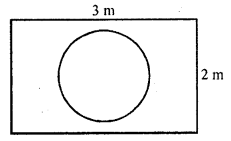Solution: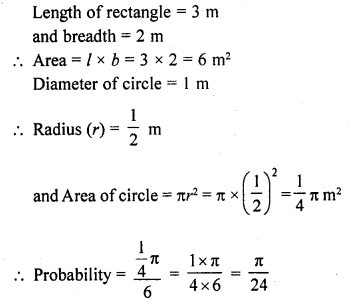Question 2.
In the accompanying diagram a fair spinner is placed at the centre O of the circle. Diameter AOB and radius OC divide the circle into three rigions labelled X, Y and Z. If ∠BOC = 45°. What is the probability that the spinner will land in the region X?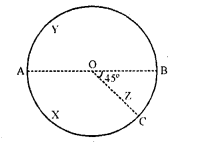Solution: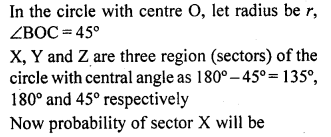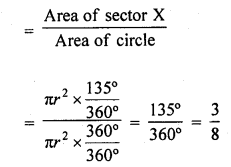Question 3.
A target shown in the figure consists of three concentric circles of radii 3, 7 and 9 cm respectively. A dart is thrown and lands on the target. What is the probability that the dart will land on the shaded region ?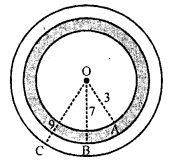Solution: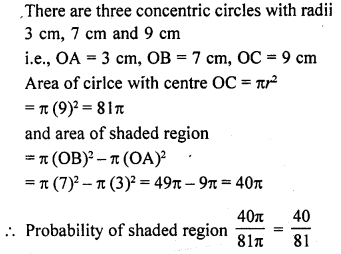Question 4.
In the figure, points A, B, C and D arc the centres of four circles that each have a radius of length one unit. If a point is selected at random from the interior o’ square ABCD. What is the probability that the point will be chosen from th; shaded region ?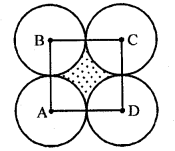Solution: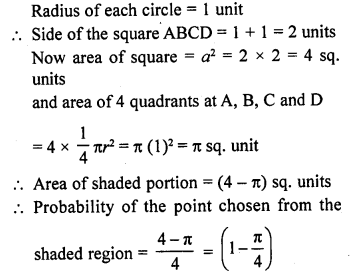Question 5.
In the figure, JKLM is a square with sides of length 6 units. Points A and B are the mid-points of sides KL and LM respectively. If a point is selected at random from the interior of the square. What is the probability that the point will be chosen from the interior of ∆JAB?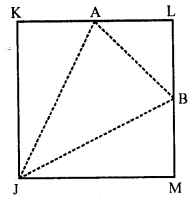Solution: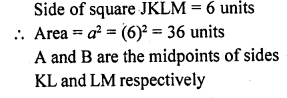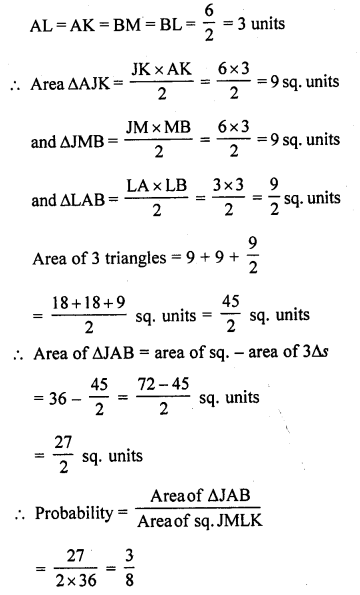Question 6.
In the figure, a square dart board is shown. The length of a side of the larger square is 1.5 times the length of a side of the smaller square. If a dart is thrown and lands on the larger square. What is the probability that it will land in the interior of the smaller square ?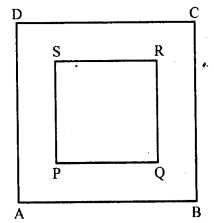Solution: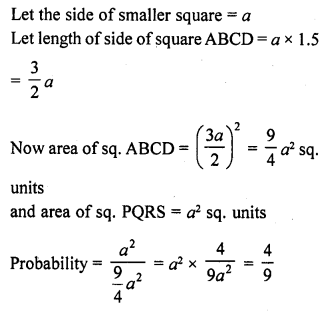Hope given RD Sharma Class 10 Solutions Chapter 16 Probability Ex 16.2 are helpful to complete your math homework.

If you have any doubts, please comment below. Learn Insta try to provide online math tutoring for you.